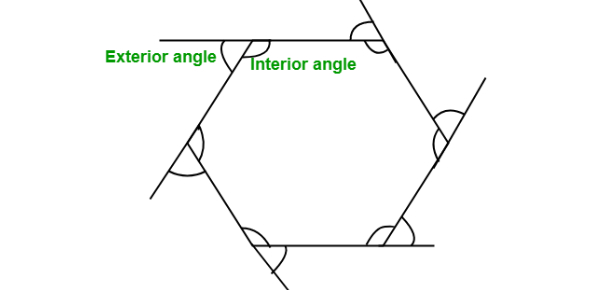# 6.2.3 Polygon Angle Sums

10 Questions | Total Attempts: 852SettingsWhen finding the angles of a polygon, we usually divide the polygon into triangles. There is a basic formula for getting the angles that was covered in class today. Take up the quiz below to help you practice on getting the angles of a polygon until it sticks. Best of luck!

• 1.
What is the interior angle sum of a decagon?
• A.

1800 degrees

• B.

1440 degrees

• C.

1080 degrees

• D.

720 degrees

• E.

360 degrees

• 2.
What is the interior angle sum of a pentagon?
• A.

360 degrees

• B.

540 degrees

• C.

720 degrees

• D.

900 degrees

• E.

1080 degrees

• 3.
What is the interior angle sum of an octagon?
• A.

360 degrees

• B.

540 degrees

• C.

720 degrees

• D.

900 degrees

• E.

1080 degrees

• 4.
What is the exterior angle sum of a pentagon?
• A.

360 degrees

• B.

540 degrees

• C.

720 degrees

• D.

900 degrees

• E.

1080 degrees

• 5.
What is the measure in degrees of one exterior angle of a regular octagon?
• A.

20

• B.

30

• C.

36

• D.

40

• E.

45

• 6.
What is the measure in degrees of one exterior angle of a regular nonegon?
• A.

20

• B.

30

• C.

40

• D.

60

• E.

90

• 7.
How many sides does a regular polygon have if each exterior angle measures 20 degrees?
• A.

18

• B.

12

• C.

10

• D.

9

• E.

8

• 8.
How many sides does a regular polygon have if each exterior angle measures 60 degrees?
• A.

10

• B.

9

• C.

8

• D.

7

• E.

6

• 9.
What is the measure of one interior angle of a regular pentagon?
• A.

108 degrees

• B.

120 degrees

• C.

135 degrees

• D.

144 degrees

• E.

150 degrees

• 10.
What is the measure of one interior angle of a regular decagon?
• A.

108

• B.

120 degrees

• C.

135 degrees

• D.

144 degrees

• E.

150 degrees

Related TopicsBack to top